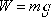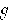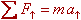weight  =  mass  times  the earth's gravitational force constant

Note: This equation is an approximation to the universal law of gravitation (which states that any two objects exert a gravitational force of attraction on each other) applicable to the case where one of the objects is an astronomical body (such as the earth) and the other is an object that is near the surface of that astronomical body. The approximation is only good near (within about 20 km in the case of the earth and a desired precision of 3 significant figures) the surface of the astronomical body.

Note: Weight is a force. It is a gravitational force. For objects near the surface of the earth, the agent of this force is the earth. Unless otherwise specified, it should be assumed that processes involving ordinary objects typically found near the surface of the earth (cars, rocks, blocks etc.) are indeed near the surface of the earth and thus subject to the weight force under discussion here withbeing the gravitational force constant applicable to the earth.

Pitfall Avoidance Note: In applying the Newton's 2nd Law equation, the weight force mis one of the forces in the sum of the forces and, hence, goes on the left side of the equation. One should never think of it as the ma that appears on the right side of the equation. Newton's 2nd Law is a statement of cause equals effect where weight is part of the cause.